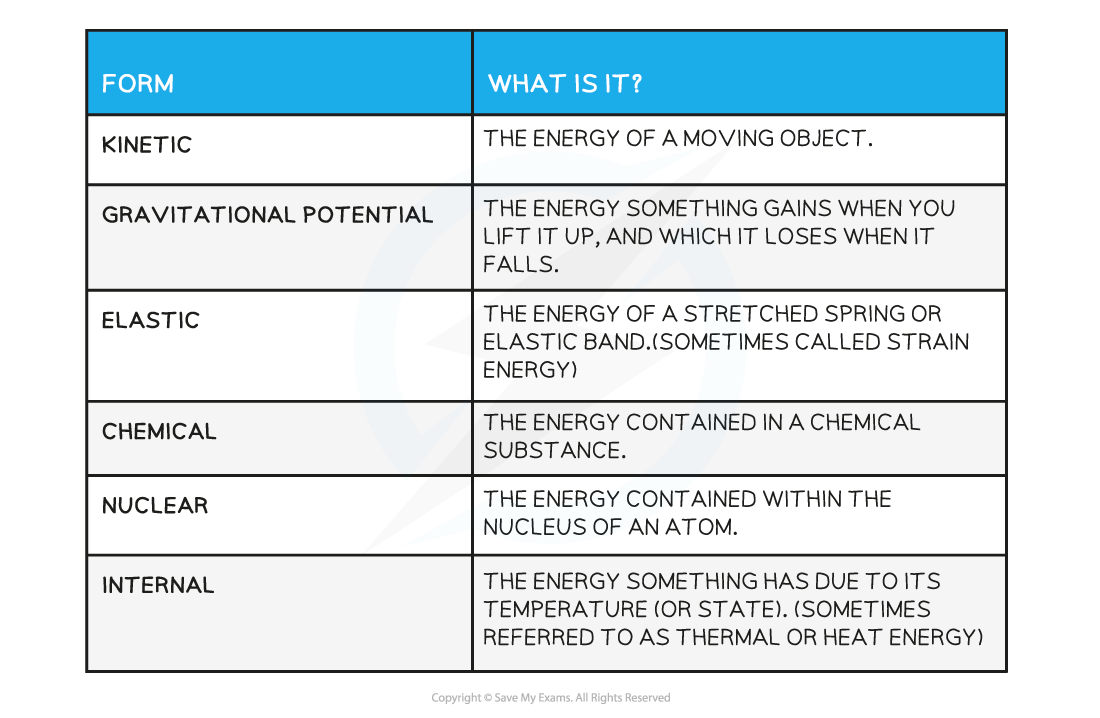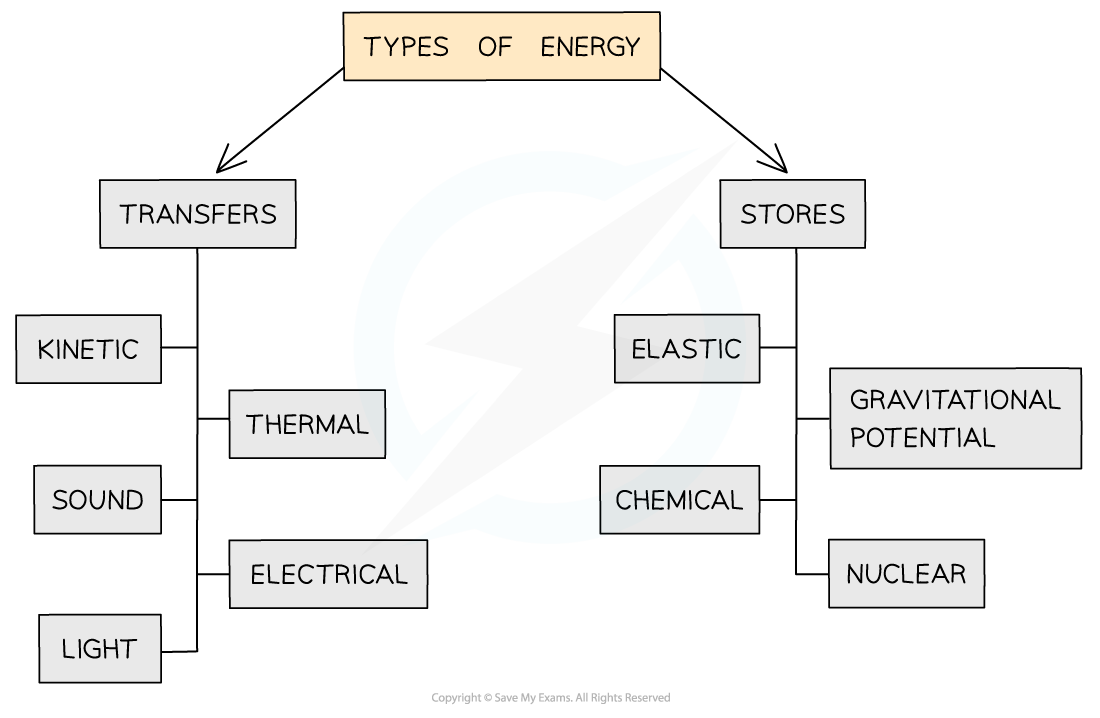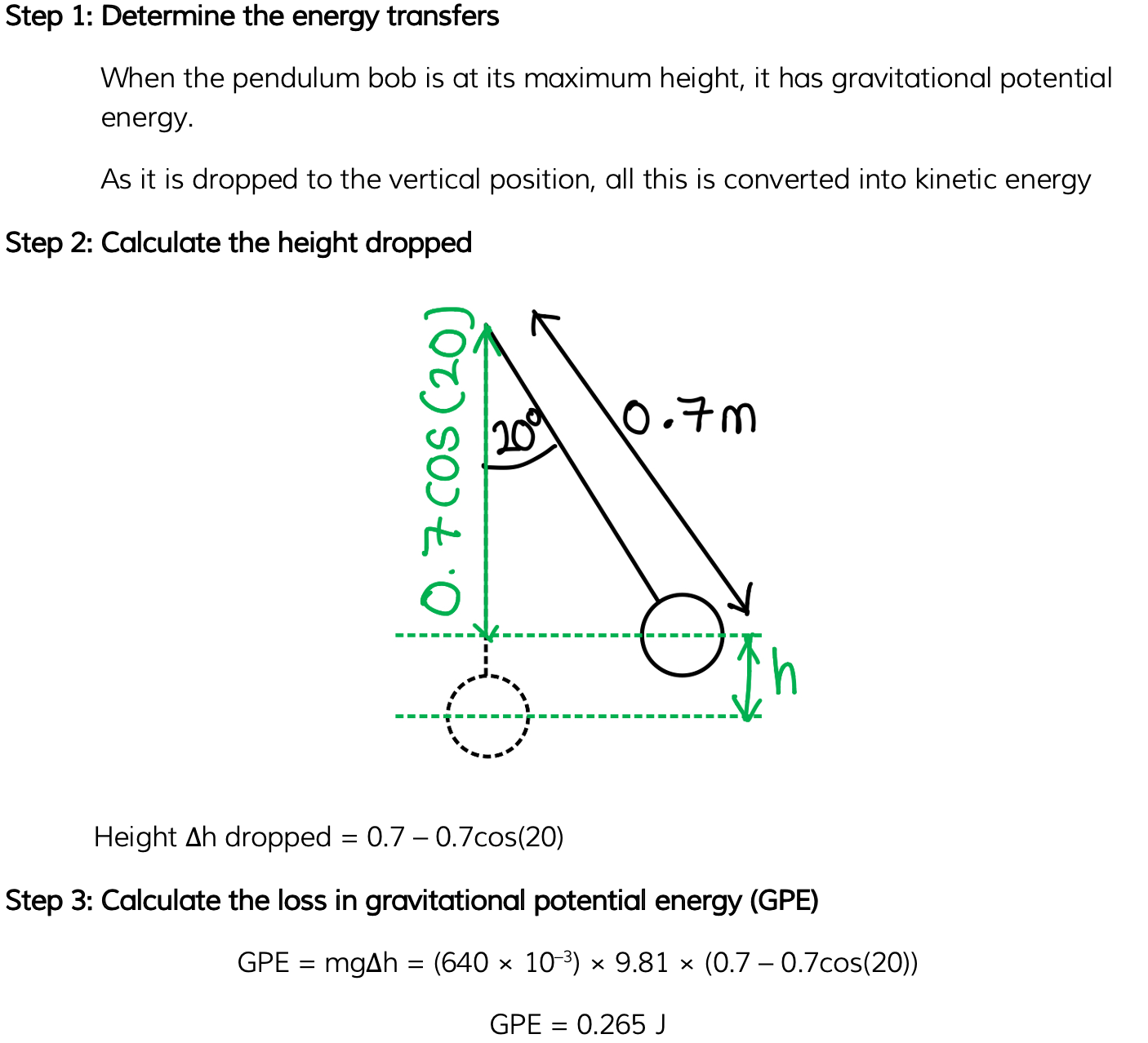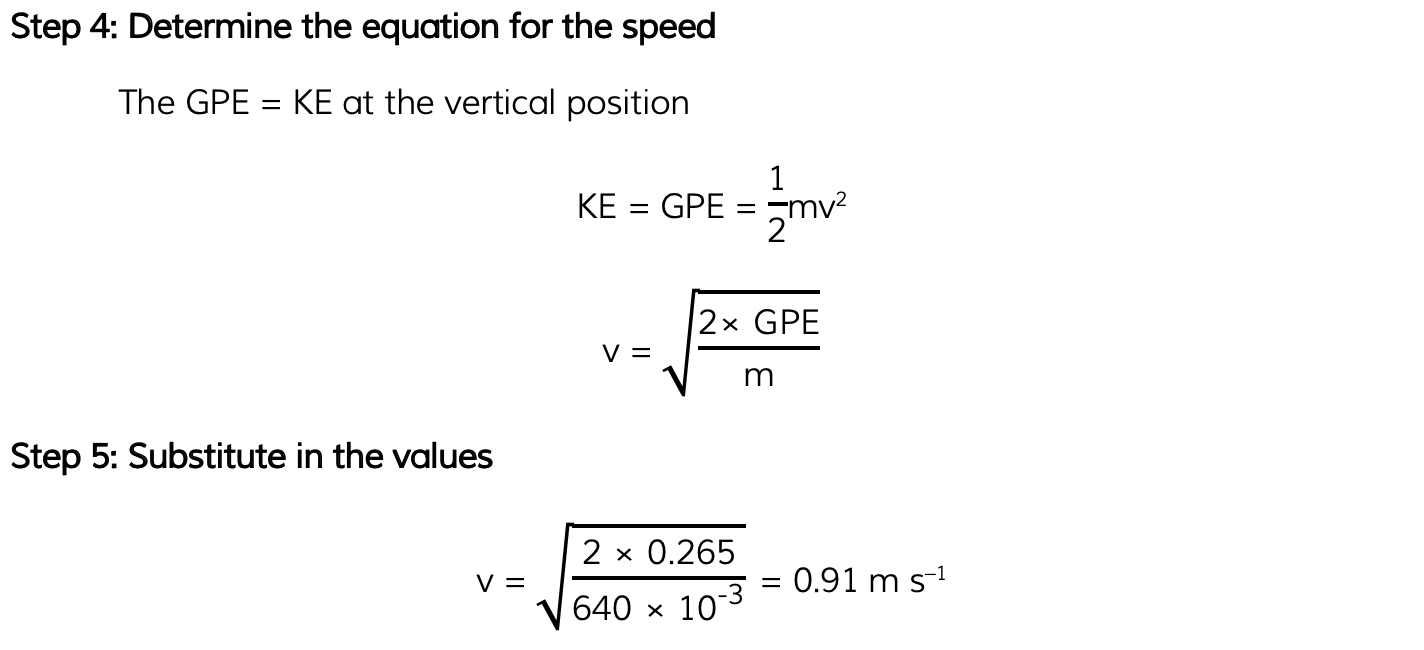# AQA A Level Physics复习笔记4.6.4 Conservation of Energy

### The Principle of Conservation of Energy

• The Principle of Conservation of Energy states that:

Energy cannot be created or destroyed, it can only be transferred from one form to another

• This means the total amount of energy in a closed system remains constant, although how much of each form there is may change

Types of EnergyEnergy types can be separated into transfers or stores

#### Energy Dissipation

• When energy is transferred from one form to another, not all the energy will end up in the desired form (or place)
• Dissipation is used to describe ways in which energy is wasted
• Any energy not transferred to useful energy stores is wasted because it is lost to the surroundings
• These are commonly in the form of thermal (heat), light or sound energy
• What counts as wasted energy depends on the system
• For example, in a television:

electrical energy ➝ light energy + sound energy + thermal energy

• Light and sound energy are useful energy transfers whereas thermal energy (from the heating up of wires) is wasted
• Another example, in a heater:

electrical energy ➝ thermal energy + sound energy

• The thermal energy is useful, whereas sound is not

#### Worked Example

The diagram shows a rollercoaster going down a track.

The rollercoaster takes the path A → B → C → D.Which statement is true about the energy changes that occur for the rollercoaster down this track?

A.     KE - GPE - GPE - KE

B.     KE - GPE - KE - GPE

C.     GPE - KE - KE - GPE

D.     GPE - KE - GPE - KE

• At point A:
• The rollercoaster is raised above the ground, therefore it has GPE
• As it travels down the track, GPE is converted to KE and the roller coaster speeds up

• At point B:
• KE is converted to GPE as the rollercoaster rises up the loop

• At point C:
• This GPE is converted back into KE as the rollercoaster travels back down the loop

• At point D:
• The flat terrain means the rollercoaster only has KE

### Applications of Energy Conservation

• Common examples of energy transfers are:
• A falling object (in a vacuum): gravitational potential energy ➝ kinetic energy
• A battery: chemical energy ➝ electrical energy ➝ light energy (if connected to a bulb)
• Horizontal mass on a spring: elastic potential energy ➝ kinetic energyEnergy transfers whilst jumping on a trampoline

• There may also be work done against resistive forces such as friction
• For example, if an object travels up a rough inclined surface, then

Loss in kinetic energy = Gain in gravitational potential energy + Work done against friction

#### Energy Equations

• The most common equations used in the conservation of energy calculations are:#### Worked Example

A simple pendulum has a mass of 640 g and a length of 0.7 m. It is pulled out to an angle of 20° from the vertical.The pendulum is released. Assuming negligible air resistance, calculate the maximum speed of the pendulum bob as it passes through the vertical position.#### Exam Tip

You may not always be given the energy transfers happening in the system in exam questions.By familiarising yourself with the transfers and stores of energy, you will be expected to relate these to the situation in question.For example, a ball rolling down a hill is transferring gravitational potential energy to kinetic energy whilst a spring converts elastic potential energy into kinetic energy.